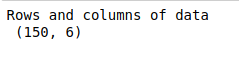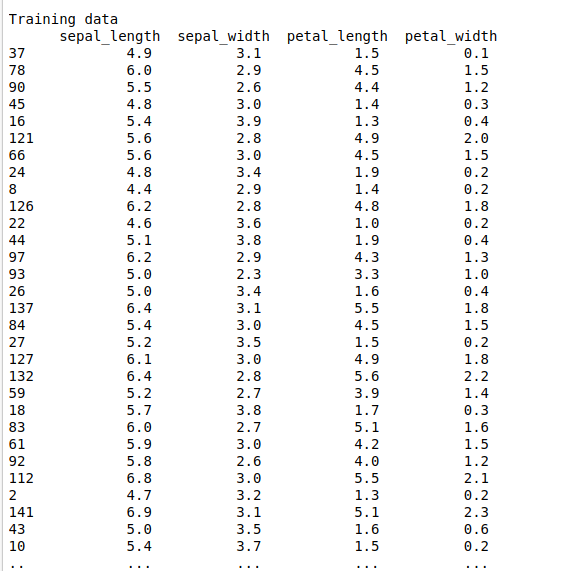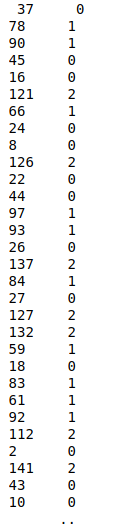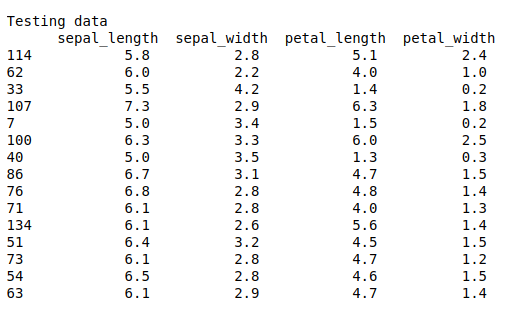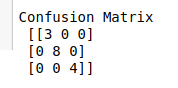• #5, First Floor, 4th Street Dr. Subbarayan Nagar Kodambakkam, Chennai-600 024 Landmark : Samiyar Madam
• pro@slogix.in
• +91- 81240 01111

### How to build Deep neural network model using keras in python?

###### Description

To build a deep neural network model for a classification problem in python.

Iris Data set.

###### Output

Confusion matrix
Classification report
Accuracy score
Precision and recall

#### process:

Define the independent and dependent variable.

Build the deep neural networks.

Initiate activation and optimizer functions according to the problem.

Split the data into train and testing set..

Fit the training set into the model.

Predict the test results using DNN.

Calculate the accuracy, precision and recall.

###### Sample Code

#import necessary libraries
import time
import random
import warnings
warnings.filterwarnings(“ignore”)
import pandas as pd
import numpy as np
import matplotlib.pyplot as plt
import seaborn as sns
from keras.models import Sequential
from keras.layers import Dense
from keras.models import Model
from sklearn.model_selection import train_test_split
from keras.utils import np_utils
from sklearn.metrics import classification_report, confusion_matrix

#load the sample data from csv file

#Make it as a data frame
df = pd.DataFrame(data)

#shape of data
print(“Rows and columns of data\n”,df.shape)
print(“\n”)

#feature selection
X = df.iloc[:,0:4]
y = df.iloc[:,5]

#Split the data into train and testing
X_train, X_test, Y_train, Y_test = train_test_split(X, y, test_size=0.1, random_state=0)

#Print training data
print(“Training data\n”,X_train,”\n”,Y_train)
print(“\n\n”)

#Print testing data
print(“Testing data\n”,X_test)
print(“\n\n”)

#make dependent variable categorical
Y_train = np_utils.to_categorical(Y_train,num_classes=3)
Y_test = np_utils.to_categorical(Y_test,num_classes=3)

batch_size = 20

#shape of input
n_cols_2 = X_train.shape

#create deep neural networks
model_2 = Sequential()

#Compile the model

#Here we train the Network.
start_time = time.time()
model_2.fit(X_train, Y_train, batch_size = batch_size, epochs = 10, verbose = 5)
end_time = time.time()
elapsed_time = end_time – start_time
print(“Time to train model: %.3f seconds” % elapsed_time)

#Evaluate the network
start_time = time.time()
score,acc = model_2.evaluate(X_test,Y_test,verbose = 2,batch_size = batch_size)
end_time = time.time()
elapsed_time = end_time – start_time
print(“Time to evaluate model: %.3f seconds” % elapsed_time)
print(“\n”)

#Predict the test results
prediction = model_2.predict(X_test)
length = len(prediction)
y_label = np.argmax(Y_test,axis=1)
predict_label = np.argmax(prediction,axis=1)

#classification report
print(“Confusion Matrix\n”,confusion_matrix(Y_test.argmax(axis=1),prediction.argmax(axis=1)))
print(“\n”)
print(“Classification Report\n”,classification_report(Y_test.argmax(axis=1),prediction.argmax(axis=1)))
print(“\n”)
accuracy = np.sum(y_label==predict_label)/length * 100
print(“Accuracy : “,accuracy)

#Precision-recall graph
plt.rcParams[“figure.figsize”] = [16,10]
import scikitplot as skplt
probas = model_2.predict_proba(X_test)
skplt.metrics.plot_precision_recall(Y_test.argmax(axis=1), probas)
plt.title(“Precision & Recall graph for DNN model”)
plt.show()

###### Screenshots